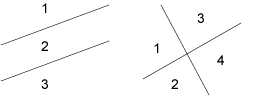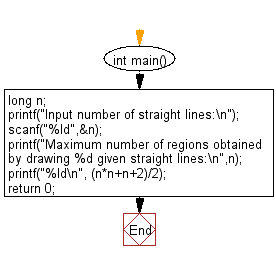﻿ C : Number of regions obtained by drawing n straight lines

# C Exercises: Create maximum number of regions obtained by drawing n given straight lines

## C Basic Declarations and Expressions: Exercise-144 with Solution

Write a C program to create the maximum number of regions obtained by drawing n given straight lines.

If you draw a straight line on a plane, the plane is divided into two regions. For example, if you pull two straight lines in parallel, you get three areas, and if you draw vertically one to the other, you get four areas.

Input:
(1 ≤ n ≤ 10,000)Sample Solution:

C Code:

``````#include <stdio.h>

int main() {
long n;

// Prompt user to input number of straight lines
printf("Input number of straight lines:\n");
scanf("%ld", &n);

// Calculate and display maximum number of regions obtained by drawing 'n' given straight lines
printf("Maximum number of regions obtained by drawing %ld given straight lines:\n", n);
printf("%ld\n", (n*n+n+2)/2);

return 0;
}
``````

Sample Output:

```Input number of straight lines:
2
Maximum number of regions obtained by drawing 2 given straight lines:
4
```

Flowchart:C programming Code Editor:

What is the difficulty level of this exercise?

Test your Programming skills with w3resource's quiz.

﻿